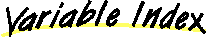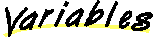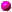Class java.awt.Rectangle
`All Packages  Class Hierarchy  This Package  Previous  Next  Index`

# Class java.awt.Rectangle

```Object
|
+----java.awt.Rectangle
```

public class Rectangle
extends Object
implements Shape, Serializable
A rectangle specifies an area in a coordinate space that is defined by the rectangle's top-left point (xy) in the coordinate space, its width, and its height.

A rectangle's `width` and `height` are public fields. The constructors that allow you to create a rectangle, and the methods that allow you to modify one, do not prevent you from setting a negative value for width or height.

A rectangle whose width or height is negative is considered empty, and all methods defined by the `Rectangle` class behave accordingly. If the rectangle is empty, then the method `isEmpty` returns `true`. No point can be contained by or inside an empty rectangle, however the values of `width` and `height` are still valid. An empty rectangle still has a location in the coordinate space, and methods that change its size or location remain valid. The behavior of methods that operate on more than one rectangle is undefined if any of the participating rectangles has a negative `width` or `height`. These methods include `intersects`, `intersection`, and `union`.

Version:
1.28, 07/01/98
Author:
Sami Shaio
Since:
JDK1.0

##height
The height of the rectangle.width
The width of the rectangle.x
The x coordinate of the rectangle.y
The y coordinate of the rectangle.

##java.awt.Rectangle()
Constructs a new rectangle whose top-left corner is at (0, 0) in the coordinate space, and whose width and height are zero.java.awt.Rectangle(Rectangle)
Constructs a new rectangle, initialized to match the values of the specificed rectangle.java.awt.Rectangle(int, int, int, int)
Constructs a new rectangle whose top-left corner is specified as (`x``y`) and whose width and height are specified by the arguments of the same name.java.awt.Rectangle(int, int)
Constructs a new rectangle whose top-left corner is at (0, 0) in the coordinate space, and whose width and height are specified by the arguments of the same name.java.awt.Rectangle(Point, Dimension)
Constructs a new rectangle whose top-left corner is specified by the `point` argument, and whose width and height are specified by the `dimension` argument.java.awt.Rectangle(Point)
Constructs a new rectangle whose top-left corner is the specified point, and whose width and height are zero.java.awt.Rectangle(Dimension)
Constructs a new rectangle whose top left corner is (0, 0) and whose width and height are specified by the `dimension` argument.

##add(int, int)
Adds a point, specified by the integer arguments `newx` and `newy`, to this rectangle.add(Point)
Adds the point `pt` to this rectangle.add(Rectangle)
Adds a rectangle to this rectangle.contains(Point)
Checks whether this rectangle contains the specified point.contains(int, int)
Checks whether this rectangle contains the point at the specified location (xy).equals(Object)
Checks whether two rectangles are equal.getBounds()
Gets the bounding rectangle of this rectangle.getLocation()
Returns the location of this rectangle.getSize()
Gets the size (width and height) of this rectangle.grow(int, int)
Grows the rectangle both horizontally and vertically.hashCode()
Returns the hashcode for this rectangle.inside(int, int)intersection(Rectangle)
Computes the intersection of this rectangle with the specified rectangle.intersects(Rectangle)
Determines whether this rectangle and the specified rectangle intersect.isEmpty()
Determines whether this rectangle is empty.move(int, int)reshape(int, int, int, int)resize(int, int)setBounds(Rectangle)
Sets the bounding rectangle of this rectangle to match the specified rectangle.setBounds(int, int, int, int)
Sets the bounding rectangle of this rectangle to the specified values for `x`, `y`, `width`, and `height`.setLocation(Point)
Moves the rectangle to the specified location.setLocation(int, int)
Moves the rectangle to the specified location.setSize(Dimension)
Sets the size of this rectangle to match the specified dimension.setSize(int, int)
Sets the size of this rectangle to the specified width and height.toString()
Returns a string representation of this rectangle and its values.translate(int, int)
Translates the rectangle the indicated distance, to the right along the x coordinate axis, and downward along the y coordinate axis.union(Rectangle)
Computes the union of this rectangle with the specified rectangle.

##height
```public int height
```
The height of the rectangle.

Since: JDK1.0width
```public int width
```
The width of the rectangle.

Since: JDK1.0.x
```public int x
```
The x coordinate of the rectangle.

Since: JDK1.0y
```public int y
```
The y coordinate of the rectangle.

Since: JDK1.0

##Rectangle
```public Rectangle()
```
Constructs a new rectangle whose top-left corner is at (0, 0) in the coordinate space, and whose width and height are zero.

Since:
JDK1.0Rectangle
```public Rectangle(Rectangle r)
```
Constructs a new rectangle, initialized to match the values of the specificed rectangle.

Parameters:
r - a rectangle from which to copy initial values.
Since:
JDK1.1Rectangle
```public Rectangle(int x,
int y,
int width,
int height)
```
Constructs a new rectangle whose top-left corner is specified as (`x``y`) and whose width and height are specified by the arguments of the same name.

Parameters:
x - the x coordinate.
y - the y coordinate.
width - the width of the rectangle.
height - the height of the rectangle.
Since:
JDK1.0Rectangle
```public Rectangle(int width,
int height)
```
Constructs a new rectangle whose top-left corner is at (0, 0) in the coordinate space, and whose width and height are specified by the arguments of the same name.

Parameters:
width - the width of the rectangle.
height - the height of the rectangle.
Since:
JDK1.0Rectangle
```public Rectangle(Point p,
Dimension d)
```
Constructs a new rectangle whose top-left corner is specified by the `point` argument, and whose width and height are specified by the `dimension` argument.

Parameters:
p - a point, the top-left corner of the rectangle.
d - a dimension, representing the width and height.
Since:
JDK1.0Rectangle
```public Rectangle(Point p)
```
Constructs a new rectangle whose top-left corner is the specified point, and whose width and height are zero.

Parameters:
p - the top left corner of the rectangle.
Since:
JDK1.0Rectangle
```public Rectangle(Dimension d)
```
Constructs a new rectangle whose top left corner is (0, 0) and whose width and height are specified by the `dimension` argument.

Parameters:
d - a dimension, specifying width and height.
Since:
JDK1.0

##add
```public void add(int newx,
int newy)
```
Adds a point, specified by the integer arguments `newx` and `newy`, to this rectangle. The resulting rectangle is the smallest rectangle that contains both the original rectangle and the specified point.

After adding a point, a call to ```contains with the added point as an argument will not necessarily return true. The contains method does not return true for points on the right or bottom edges of a rectangle. Therefore if the added point falls on the left or bottom edge of the enlarged rectangle, contains will return false for that point. ```

``` ```

``` Parameters: newx - the x coordinate of the new point. newy - the y coordinate of the new point. Since: JDK1.0 ```
``` ```
```add public void add(Point pt) Adds the point pt to this rectangle. The resulting rectangle is the smallest rectangle that contains both the original rectangle and the specified point. After adding a point, a call to contains with the added point as an argument will not necessarily return true. The contains method does not return true for points on the right or bottom edges of a rectangle. Therefore if the added point falls on the left or bottom edge of the enlarged rectangle, contains will return false for that point. Parameters: pt - the new point to add to the rectangle. Since: JDK1.0add public void add(Rectangle r) Adds a rectangle to this rectangle. The resulting rectangle is the union of the two rectangles. Parameters: a - rectangle. Since: JDK1.0contains public boolean contains(Point p) Checks whether this rectangle contains the specified point. Parameters: p - the point (location) to test. Returns: true if the point (x, y) is inside this rectangle; false otherwise. Since: JDK1.1contains public boolean contains(int x, int y) Checks whether this rectangle contains the point at the specified location (x, y). Parameters: x - the x coordinate. y - the y coordinate. Returns: true if the point (x, y) is inside this rectangle; false otherwise. Since: JDK1.1equals public boolean equals(Object obj) Checks whether two rectangles are equal. The result is true if and only if the argument is not null and is a Rectangle object that has the same top-left corner, width, and height as this rectangle. Parameters: obj - the object to compare with. Returns: true if the objects are equal; false otherwise. Overrides: equals in class Object Since: JDK1.0getBounds public java.awt.Rectangle getBounds() Gets the bounding rectangle of this rectangle. This method is included for completeness, to parallel the getBounds method of Component. Returns: a new rectangle, equal to the bounding rectangle for this rectangle. Since: JDK1.1 See Also: getBoundsgetLocation public java.awt.Point getLocation() Returns the location of this rectangle. This method is included for completeness, to parallel the getLocation method of Component. Since: JDK1.1 See Also: getLocationgetSize public java.awt.Dimension getSize() Gets the size (width and height) of this rectangle. This method is included for completeness, to parallel the getSize method of Component. Returns: a dimension, representing the size. Since: JDK1.1 See Also: getSizegrow public void grow(int h, int v) Grows the rectangle both horizontally and vertically. This method modifies the rectangle so that it is h units larger on both the left and right side, and v units larger at both the top and bottom. The new rectangle has (x - h, y - v) as its top-left corner, a width of width + 2h, and a height of height + 2v. If negative values are supplied for h and v, the size of the rectangle decreases accordingly. The grow method does not check whether the resulting values of width and height are non-negative. Parameters: h - the horizontal expansion. v - the vertical expansion. Since: JDK1.0hashCode public int hashCode() Returns the hashcode for this rectangle. Returns: the hashcode for this rectangle. Overrides: hashCode in class Object Since: JDK1.0inside public boolean inside(int x, int y) Note: inside() is deprecated. As of JDK version 1.1, replaced by contains(int, int).intersection public java.awt.Rectangle intersection(Rectangle r) Computes the intersection of this rectangle with the specified rectangle. Returns a new rectangle that represents the intersection of the two rectangles. Parameters: r - a rectangle. Returns: the largest rectangle contained in both the specified rectangle and in this rectangle. Since: JDK1.0intersects public boolean intersects(Rectangle r) Determines whether this rectangle and the specified rectangle intersect. Two rectangles intersect if their intersection is nonempty. Parameters: r - a rectangle. Returns: true if the specified rectangle and this rectangle insersect; false otherwise. Since: JDK1.0isEmpty public boolean isEmpty() Determines whether this rectangle is empty. A rectangle is empty if its width or its height is less than or equal to zero. Returns: true if this rectangle is empty; false otherwise. Since: JDK1.0move public void move(int x, int y) Note: move() is deprecated. As of JDK version 1.1, replaced by setLocation(int, int).reshape public void reshape(int x, int y, int width, int height) Note: reshape() is deprecated. As of JDK version 1.1, replaced by setBounds(int, int, int, int).resize public void resize(int width, int height) Note: resize() is deprecated. As of JDK version 1.1, replaced by setSize(int, int).setBounds public void setBounds(Rectangle r) Sets the bounding rectangle of this rectangle to match the specified rectangle. This method is included for completeness, to parallel the setBounds method of Component. Parameters: r - a rectangle. Since: JDK1.1 See Also: setBounds(java.awt.Rectangle)setBounds public void setBounds(int x, int y, int width, int height) Sets the bounding rectangle of this rectangle to the specified values for x, y, width, and height. This method is included for completeness, to parallel the setBounds method of Component. Parameters: x - the new x coordinate for the top-left corner of this rectangle. y - the new y coordinate for the top-left corner of this rectangle. width - the new width for this rectangle. height - the new height for this rectangle. Since: JDK1.1 See Also: setBounds(int, int, int, int)setLocation public void setLocation(Point p) Moves the rectangle to the specified location. This method is included for completeness, to parallel the setLocation method of Component. Parameters: p - the new location for the point. Since: JDK1.1 See Also: setLocation(java.awt.Point)setLocation public void setLocation(int x, int y) Moves the rectangle to the specified location. This method is included for completeness, to parallel the setLocation method of Component. Parameters: x - the x coordinate of the new location. y - the y coordinate of the new location. Since: JDK1.1 See Also: setLocation(int, int)setSize public void setSize(Dimension d) Sets the size of this rectangle to match the specified dimension. This method is included for completeness, to parallel the setSize method of Component. Parameters: d - the new size for the Dimension object Since: JDK1.1 See Also: setSize(java.awt.Dimension)setSize public void setSize(int width, int height) Sets the size of this rectangle to the specified width and height. This method is included for completeness, to parallel the setSize method of Component. Parameters: width - the new width for this rectangle object. height - the new height for this rectangle object. Since: JDK1.1 See Also: setSize(int, int)toString public java.lang.String toString() Returns a string representation of this rectangle and its values. Returns: a string representation of this rectangle. Overrides: toString in class Object Since: JDK1.0translate public void translate(int x, int y) Translates the rectangle the indicated distance, to the right along the x coordinate axis, and downward along the y coordinate axis. Parameters: dx - the distance to move the rectangle along the x axis. dy - the distance to move the rectangle along the y axis. Since: JDK1.0 See Also: setLocation(int, int), setLocation(java.awt.Point)union public java.awt.Rectangle union(Rectangle r) Computes the union of this rectangle with the specified rectangle. Returns a new rectangle that represents the union of the two rectangles. Parameters: r - a rectangle. Returns: the smallest rectangle containing both the specified rectangle and this rectangle. Since: JDK1.0 All Packages Class Hierarchy This Package Previous Next Index ```